## 5.5 Reduction to the absurd.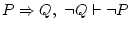This is a very useful technique. Validity ofis proved with: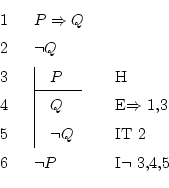What has to be achieved here is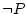, which is the negation of something, so the rule which will help is the negation introduction, also known as reduction to the absurd.

The way to act will be to suppose the contrary of(which is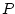) and find a contradiction (any). Supposingwill lead us to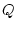(by implication elimination), and, as we also have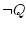, we can apply the rule. Thisshould be inserted in the current subdemonstration with the iteration rule, so that it is together with theand inside the subdemonstration. Everything which is inside the subdemonstration is consequence of, so it is important to see that bothandalso are.

For the negation introduction, the way to justify this rule is putting the line number of where does the (wrong) supposition start, and the numbers of the two lines where we saw the contradiction. The conclusion of this rule is the contrary of what we just supposed, in this case, so we can finish the derivation here.

This reasoning is actually made without much thinking. In words it would be something like: of course that, since if it werethen, and you say that, so it can't be that''.

Daniel Clemente Laboreo 2005-05-17